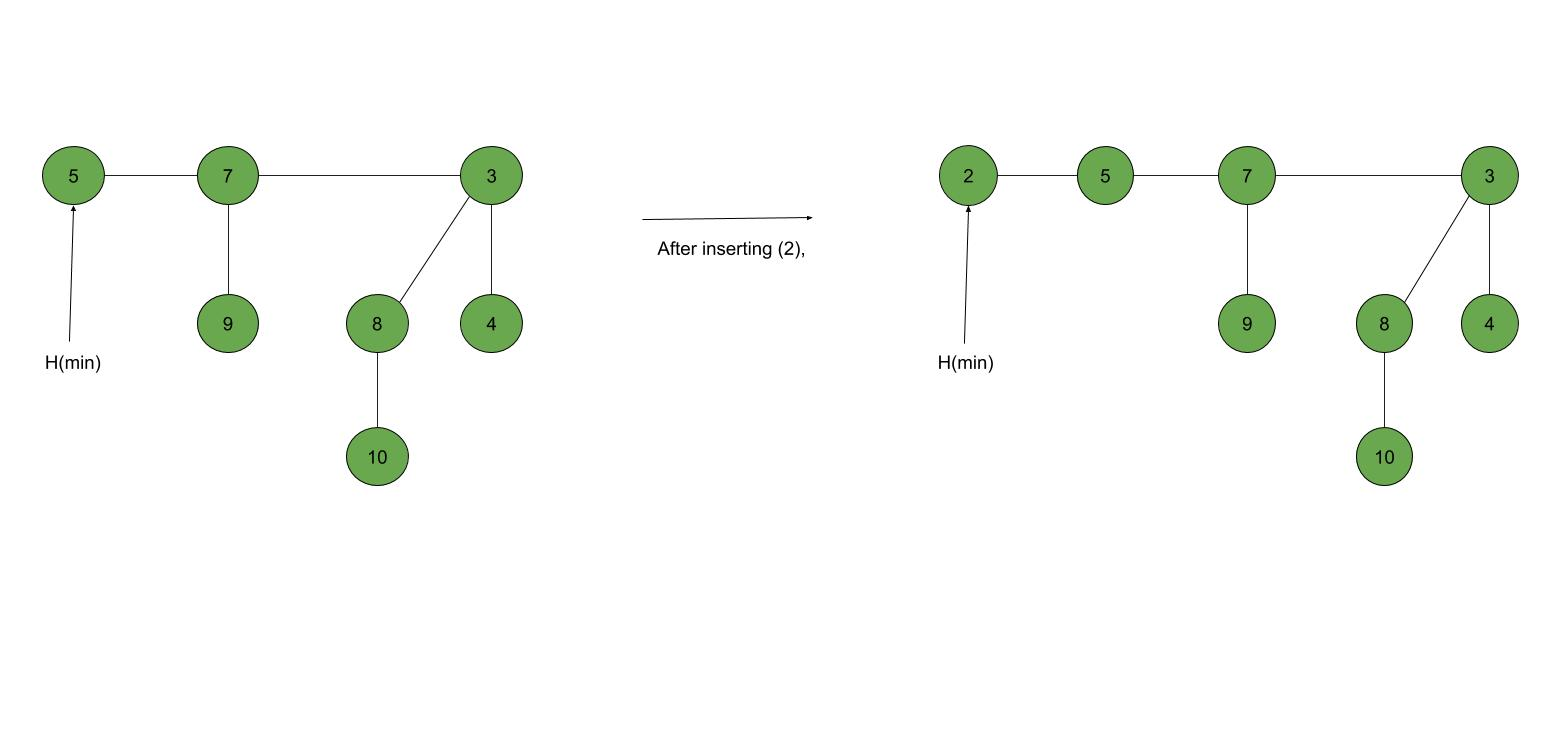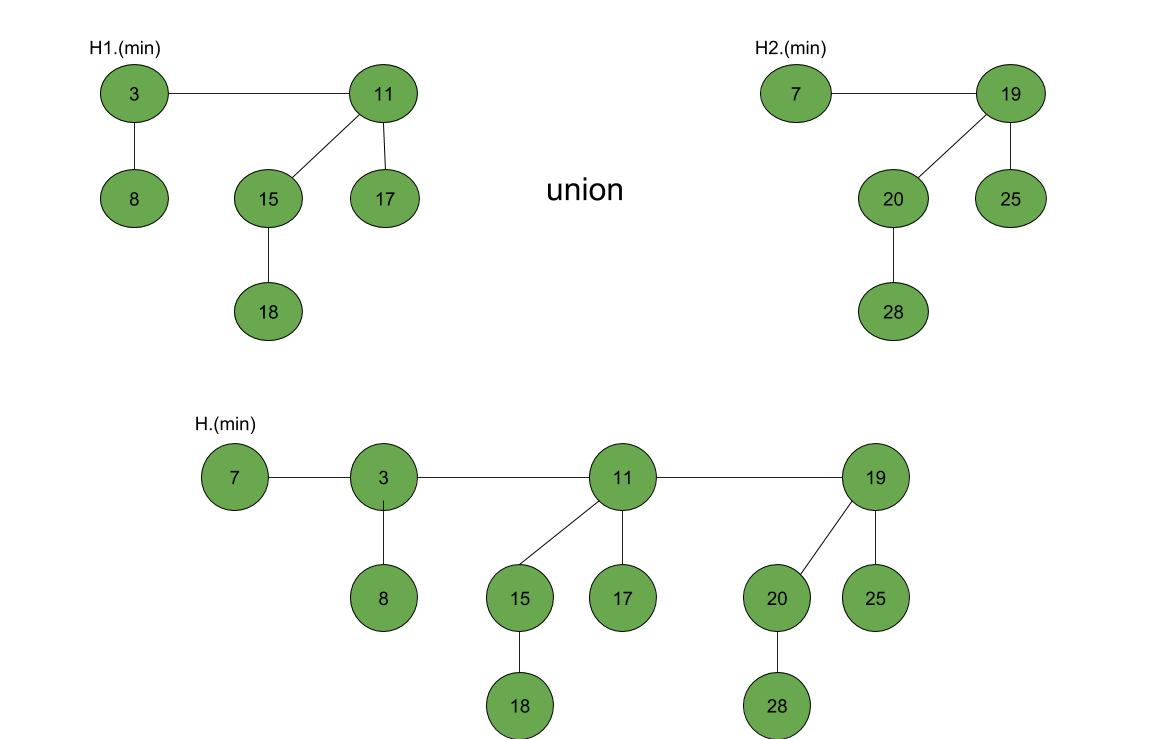Open in App
Not now

# Fibonacci Heap – Insertion and Union

• Difficulty Level : Medium
• Last Updated : 06 Sep, 2022

Fibonacci Heap is a collection of trees with min-heap or max-heap property. In Fibonacci Heap, trees can have any shape even all trees can be single nodes (This is unlike Binomial Heap where every tree has to be a Binomial Tree). In this article, we will discuss Insertion and Union operation on Fibonacci Heap.

Prerequisites: Fibonacci Heap (Introduction)

Insertion: To insert a node in a Fibonacci heap H, the following algorithm is followed:

1. Create a new node ‘x’.
2. Check whether heap H is empty or not.
3. If H is empty then:
• Make x as the only node in the root list.
• Set H(min) pointer to x.
4. Else:
• Insert x into root list and update H(min).

Example:Union: Union of two Fibonacci heaps H1 and H2 can be accomplished as follows:

1. Join root lists of Fibonacci heaps H1 and H2 and make a single Fibonacci heap H.
2. If H1(min) < H2(min) then:
• H(min) = H1(min).
3. Else:
• H(min) = H2(min).

Example:Following is a program to demonstrate building and inserting in a Fibonacci heap:

## C++

 `// C++ program to demonstrate building` `// and inserting in a Fibonacci heap` `#include ` `#include ` `#include ` `using` `namespace` `std;`   `struct` `node {` `    ``node* parent;` `    ``node* child;` `    ``node* left;` `    ``node* right;` `    ``int` `key;` `};`   `// Creating min pointer as "mini"` `struct` `node* mini = NULL;`   `// Declare an integer for number of nodes in the heap` `int` `no_of_nodes = 0;`   `// Function to insert a node in heap` `void` `insertion(``int` `val)` `{` `    ``struct` `node* new_node = (``struct` `node*)``malloc``(``sizeof``(``struct` `node));` `    ``new_node->key = val;` `    ``new_node->parent = NULL;` `    ``new_node->child = NULL;` `    ``new_node->left = new_node;` `    ``new_node->right = new_node;` `    ``if` `(mini != NULL) {` `        ``(mini->left)->right = new_node;` `        ``new_node->right = mini;` `        ``new_node->left = mini->left;` `        ``mini->left = new_node;` `        ``if` `(new_node->key < mini->key)` `            ``mini = new_node;` `    ``}` `    ``else` `{` `        ``mini = new_node;` `    ``}` `}`   `// Function to display the heap` `void` `display(``struct` `node* mini)` `{` `    ``node* ptr = mini;` `    ``if` `(ptr == NULL)` `        ``cout << ``"The Heap is Empty"` `<< endl;`   `    ``else` `{` `        ``cout << ``"The root nodes of Heap are: "` `<< endl;` `        ``do` `{` `            ``cout << ptr->key;` `            ``ptr = ptr->right;` `            ``if` `(ptr != mini) {` `                ``cout << ``"-->"``;` `            ``}` `        ``} ``while` `(ptr != mini && ptr->right != NULL);` `        ``cout << endl` `             ``<< ``"The heap has "` `<< no_of_nodes << ``" nodes"` `<< endl;` `    ``}` `}` `// Function to find min node in the heap` `void` `find_min(``struct` `node* mini)` `{` `    ``cout << ``"min of heap is: "` `<< mini->key << endl;` `}`     `// Driver code` `int` `main()` `{`   `    ``no_of_nodes = 7;` `    ``insertion(4);` `    ``insertion(3);` `    ``insertion(7);` `    ``insertion(5);` `    ``insertion(2);` `    ``insertion(1);` `    ``insertion(10);`   `    ``display(mini);`   `    ``find_min(mini);`   `    ``return` `0;` `}`

## Python3

 `# Python program to demonstrate building` `# and inserting in a Fibonacci heap`     `class` `node:` `    ``def` `__init__(``self``):` `        ``self``.parent ``=` `None`  `# Assign data` `        ``self``.child ``=` `None`  `# Initialize next as null` `        ``self``.left ``=` `None` `        ``self``.right ``=` `None` `        ``self``.key ``=` `-``1`     `# Creating min pointer as "mini"` `mini ``=` `node()`   `# Declare an integer for number of nodes in the heap` `no_of_nodes ``=` `0`   `# Function to insert a node in heap`     `def` `insertion(val):` `    ``new_node ``=` `node()` `    ``new_node.key ``=` `val` `    ``new_node.parent ``=` `None` `    ``new_node.child ``=` `None` `    ``new_node.left ``=` `new_node` `    ``new_node.right ``=` `new_node`   `    ``global` `mini` `    ``if` `mini.key !``=` `-``1``:` `        ``(mini.left).right ``=` `new_node` `        ``new_node.right ``=` `mini` `        ``new_node.left ``=` `mini.left` `        ``mini.left ``=` `new_node` `        ``if` `(new_node.key < mini.key):` `            ``mini ``=` `new_node` `    ``else``:` `        ``mini ``=` `new_node`   `# Function to display the heap`     `def` `display(mini):` `    ``ptr ``=` `mini` `    ``if` `(ptr ``=``=` `None``):` `        ``print``(``"The Heap is Empty"``)` `    ``else``:`   `        ``print``(``"The root nodes of Heap are: "``)` `        ``print``(ptr.key, end``=``"")` `        ``ptr ``=` `ptr.right` `        ``if``(ptr !``=` `mini):` `            ``print``(``"-->"``, end``=``"")`   `        ``while` `ptr !``=` `mini ``and` `ptr.right !``=` `None``:` `            ``print``(ptr.key, end``=``"")` `            ``ptr ``=` `ptr.right` `            ``if` `ptr !``=` `mini:` `                ``print``(``"-->"``, end``=``"")` `        ``print``()` `        ``print``(f``"The heap has {no_of_nodes} nodes"``)`   `# Function to find min node in the heap`     `def` `find_min(mini):` `    ``print``(f``"min of heap is: {mini.key}"``)`     `# Driver code` `no_of_nodes ``=` `7` `insertion(``4``)` `insertion(``3``)` `insertion(``7``)` `insertion(``5``)` `insertion(``2``)` `insertion(``1``)` `insertion(``10``)`   `display(mini)`   `find_min(mini)`   `# The code is contributed by Gautam goel (gautamgoel962)`

Output

```The root nodes of Heap are:
1-->2-->3-->4-->7-->5-->10
The heap has 7 nodes
min of heap is: 1
```

My Personal Notes arrow_drop_up
Related Articles